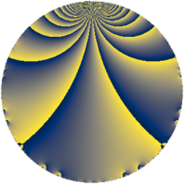Properties

 Label 7800.2.a.baLevel $7800$ Weight $2$ Character orbit 7800.a Self dual yes Analytic conductor $62.283$ Analytic rank $1$ Dimension $2$ CM no Inner twists $1$

Related objects

Newspace parameters

 Level: $$N$$ $$=$$ $$7800 = 2^{3} \cdot 3 \cdot 5^{2} \cdot 13$$ Weight: $$k$$ $$=$$ $$2$$ Character orbit: $$[\chi]$$ $$=$$ 7800.a (trivial)

Newform invariants

 Self dual: yes Analytic conductor: $$62.2833135766$$ Analytic rank: $$1$$ Dimension: $$2$$ Coefficient field: $$\Q(\sqrt{2})$$ Defining polynomial: $$x^{2} - 2$$ Coefficient ring: $$\Z[a_1, \ldots, a_{7}]$$ Coefficient ring index: $$1$$ Twist minimal: yes Fricke sign: $$1$$ Sato-Tate group: $\mathrm{SU}(2)$

$q$-expansion

Coefficients of the $$q$$-expansion are expressed in terms of $$\beta = \sqrt{2}$$. We also show the integral $$q$$-expansion of the trace form.

 $$f(q)$$ $$=$$ $$q + q^{3} + ( -1 + \beta ) q^{7} + q^{9} +O(q^{10})$$ $$q + q^{3} + ( -1 + \beta ) q^{7} + q^{9} + ( -1 - \beta ) q^{11} + q^{13} + ( -1 + 2 \beta ) q^{17} -2 q^{19} + ( -1 + \beta ) q^{21} + ( -2 - 2 \beta ) q^{23} + q^{27} + ( -3 + 4 \beta ) q^{29} + ( 3 - \beta ) q^{31} + ( -1 - \beta ) q^{33} + ( 2 - 4 \beta ) q^{37} + q^{39} -4 \beta q^{41} + ( 2 - 6 \beta ) q^{43} + ( -5 - 5 \beta ) q^{47} + ( -4 - 2 \beta ) q^{49} + ( -1 + 2 \beta ) q^{51} + ( 3 - 2 \beta ) q^{53} -2 q^{57} + ( -5 + \beta ) q^{59} + ( 1 + 2 \beta ) q^{61} + ( -1 + \beta ) q^{63} + ( -5 - 3 \beta ) q^{67} + ( -2 - 2 \beta ) q^{69} + ( -2 + 4 \beta ) q^{71} + ( 2 + 8 \beta ) q^{73} - q^{77} + ( -6 + 2 \beta ) q^{79} + q^{81} + ( -1 + 9 \beta ) q^{83} + ( -3 + 4 \beta ) q^{87} + ( -1 + \beta ) q^{91} + ( 3 - \beta ) q^{93} + ( 2 + 12 \beta ) q^{97} + ( -1 - \beta ) q^{99} +O(q^{100})$$ $$\operatorname{Tr}(f)(q)$$ $$=$$ $$2 q + 2 q^{3} - 2 q^{7} + 2 q^{9} + O(q^{10})$$ $$2 q + 2 q^{3} - 2 q^{7} + 2 q^{9} - 2 q^{11} + 2 q^{13} - 2 q^{17} - 4 q^{19} - 2 q^{21} - 4 q^{23} + 2 q^{27} - 6 q^{29} + 6 q^{31} - 2 q^{33} + 4 q^{37} + 2 q^{39} + 4 q^{43} - 10 q^{47} - 8 q^{49} - 2 q^{51} + 6 q^{53} - 4 q^{57} - 10 q^{59} + 2 q^{61} - 2 q^{63} - 10 q^{67} - 4 q^{69} - 4 q^{71} + 4 q^{73} - 2 q^{77} - 12 q^{79} + 2 q^{81} - 2 q^{83} - 6 q^{87} - 2 q^{91} + 6 q^{93} + 4 q^{97} - 2 q^{99} + O(q^{100})$$

Embeddings

For each embedding $$\iota_m$$ of the coefficient field, the values $$\iota_m(a_n)$$ are shown below.

For more information on an embedded modular form you can click on its label.

Label $$\iota_m(\nu)$$ $$a_{2}$$ $$a_{3}$$ $$a_{4}$$ $$a_{5}$$ $$a_{6}$$ $$a_{7}$$ $$a_{8}$$ $$a_{9}$$ $$a_{10}$$
1.1
 −1.41421 1.41421
0 1.00000 0 0 0 −2.41421 0 1.00000 0
1.2 0 1.00000 0 0 0 0.414214 0 1.00000 0
 $$n$$: e.g. 2-40 or 990-1000 Significant digits: Format: Complex embeddings Normalized embeddings Satake parameters Satake angles

Atkin-Lehner signs

$$p$$ Sign
$$2$$ $$1$$
$$3$$ $$-1$$
$$5$$ $$1$$
$$13$$ $$-1$$

Inner twists

This newform does not admit any (nontrivial) inner twists.

Twists

By twisting character orbit
Char Parity Ord Mult Type Twist Min Dim
1.a even 1 1 trivial 7800.2.a.ba yes 2
5.b even 2 1 7800.2.a.z 2

By twisted newform orbit
Twist Min Dim Char Parity Ord Mult Type
7800.2.a.z 2 5.b even 2 1
7800.2.a.ba yes 2 1.a even 1 1 trivial

Hecke kernels

This newform subspace can be constructed as the intersection of the kernels of the following linear operators acting on $$S_{2}^{\mathrm{new}}(\Gamma_0(7800))$$:

 $$T_{7}^{2} + 2 T_{7} - 1$$ $$T_{11}^{2} + 2 T_{11} - 1$$ $$T_{17}^{2} + 2 T_{17} - 7$$ $$T_{19} + 2$$

Hecke characteristic polynomials

$p$ $F_p(T)$
$2$ $$T^{2}$$
$3$ $$( -1 + T )^{2}$$
$5$ $$T^{2}$$
$7$ $$-1 + 2 T + T^{2}$$
$11$ $$-1 + 2 T + T^{2}$$
$13$ $$( -1 + T )^{2}$$
$17$ $$-7 + 2 T + T^{2}$$
$19$ $$( 2 + T )^{2}$$
$23$ $$-4 + 4 T + T^{2}$$
$29$ $$-23 + 6 T + T^{2}$$
$31$ $$7 - 6 T + T^{2}$$
$37$ $$-28 - 4 T + T^{2}$$
$41$ $$-32 + T^{2}$$
$43$ $$-68 - 4 T + T^{2}$$
$47$ $$-25 + 10 T + T^{2}$$
$53$ $$1 - 6 T + T^{2}$$
$59$ $$23 + 10 T + T^{2}$$
$61$ $$-7 - 2 T + T^{2}$$
$67$ $$7 + 10 T + T^{2}$$
$71$ $$-28 + 4 T + T^{2}$$
$73$ $$-124 - 4 T + T^{2}$$
$79$ $$28 + 12 T + T^{2}$$
$83$ $$-161 + 2 T + T^{2}$$
$89$ $$T^{2}$$
$97$ $$-284 - 4 T + T^{2}$$